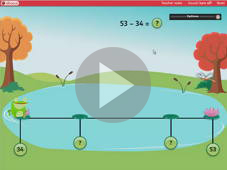Counting up to find a difference Worksheet Answers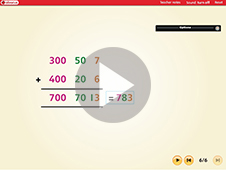Expanded addition (version 1) Worksheet Answers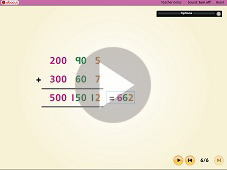Expanded addition (version 2) Worksheet Answers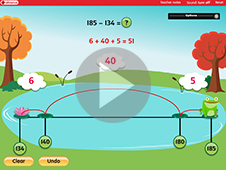Counting up to find a difference Worksheet Answers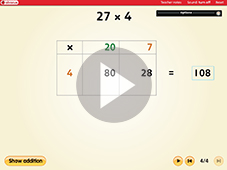Using the grid method to multiply Worksheet Answers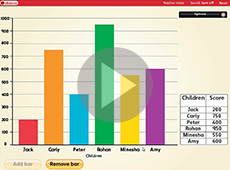Using a bar chart to show information Worksheet Answers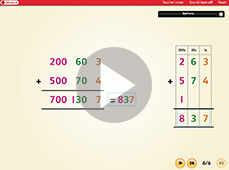Adding 3 digit numbers using a written method Worksheet Answers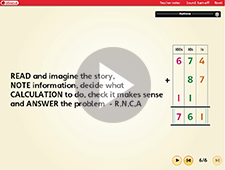Choosing a method to solve addition problems Worksheet Answers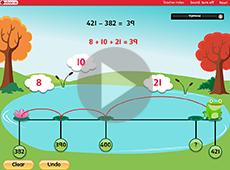Solve subtraction problems by counting up Worksheet Answers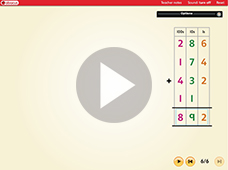Adding 3 digit numbers using column addition Worksheet Answers